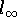Fixed Point Theory and Applications

#Fixed Point Theory and Applications

## Chapter 1. The Contraction Principle

1.
Historical Notes
2.
The Contraction Principle in Complete Metric Spaces
3.
Applications
3.1
Differential Equations
3.2
Implicit Theorem
3.3
Holomorphic Mappings
3.4
Perron-Frobenius Theorem
3.5
Fractal sets: The Cantor set

4.
Menger Spaces

## Chapter 2. Nonexpansive Mappings in Hilbert Spaces

1.
Some Examples
2.
Classical Theorems
2.1
Demi-Closeness Principle
2.2
Existence of Fixed Point

3.
Semi-group of Nonexpansive Mappings
3.1
Basic Definitions
3.2
Generator
3.3
Accretivity, Monotone Operators
3.4
Resolvent
3.5
Theorems
3.6
Example:4.
Commuting Families
5.
Uniformly Lipschitzian Maps
6.
Applications
6.1
Periodic Solutions
6.2
Forcing

## Chapter 3. Nonexpansive Mappings in Banach Spaces

1.
Classical Counter Examples
1.1,1.21.3
Alspach's counter example

2.
Uniformly Convex and Uniformly Smooth Banach Spaces
2.1
Basic Definitions
2.2
Examples
2.3
Properties
2.4
Scaling of the Unit Ball

3.
Fixed Point Theorems
3.1
Demi-Closeness Principle
3.2
Browder-Gohde Theorem
3.3
Contraction of Type-()
3.4
Acretive Operators
3.5
Asymptotic Center Technique
3.6
Minimal points and Optimal Points

4.
Normal Structure Property
4.1
Basic Definitions and Examples
4.2
Characterizations: Brodskii-Milman-Landes
4.3
Some Counter Examples
4.4
Spaces with Normal Structure Property
4.5
Normal Structure Property in Spaces with Bases

5.
Ultraproduct Techniques
5.1
Basic Definitions
5.2
Basic Results in Ultraproduct Language
5.3
Fixed Point Theorems
5.3.1
Uniformly Convex Spaces
5.3.2
Lin's results and its Extensions
5.3.3
Maurey's Fixed Point Theorems

6.
Lattice Banach Spaces
6.1
Remarks on Maurey's-Fixed point Theorem
6.2
Borwein-Sim's Fixed Point Theorem

7.-Fixed Point Property
7.1
Examples and Counter Examples
7.2-Fixed Point Property
7.3-Normal Structure Property
7.4
in Lattice Banach Spaces

## Chapter 4. Orbit, Omega-set

1.
Basic Definitions
2.
Compact Orbit
3.
Classical Techniques in Hilbert space
4.
Periodic Points
5.
Iterative Processes
6.
Applications
6.1
Atractors
6.2
Chaos

## Chapter 5. Ergodic Theorems

1.
Classical Linear results
2.
Baillon's Theorem
3.
Extensions: Amenable Semi-Group
4.
Asymptotic Behavior
5.
Examples

## Chapter 7. Non-classical Banach Spaces

1.
Orlicz spaces
2.
James' spaces
3.
Tsirelson' spaces

## Chapter 8. Metric Spaces

1.
Basic Definitions
2.
Examples
2.1
Unit Ball of2.2
Unit Ball of the Hilbert space

3.
Nonexpansive Mappings in Metric Spaces
3.1
Convexity Structures in Metric Spaces
3.2
Normal Structure Property in Metric Spaces
3.3
Kirk's analogue in Metric Spaces
3.4
Holomorphic Maps on the Unit Ball of the Hilbert space

4.
Hyperconvex Metric Spaces
4.1
Basic Definitions
4.2
Basic Properties
4.3
Fixed Point Theorems

## Chapter 9. Measure of Non-compactness

1.
Basic Definitions
2.
Examples
3.
Condensing Mappings
4.
Approximation
5.
Applications

## Chapter 10. Caristi's Fixed Point Theorem

1.
Caristi's Fixed Point Theorem
2.
Ekeland's Principle
3.
Equivalent Theorems
4.
Normal Solvability
5.
Examples and Applications

## Chapter 13. Generalized Structures

1.
Definition of an Ordered Set
2.
Tarski's Fixed point Theorem
3.
Applications
3.1
Godel's Theory
3.2
Graph Theory
3.3
Logic Programming

4.
Generalized Metric Spaces
4.1
Basic Definitions
4.2
Examples
4.3
Hyperconvex Generalized Metric Spaces
4.4
Fixed Point Theorems in Generalized Metric Spaces
4.5
Fixed Point Theorems for Families
4.6
Product Spaces
4.7
Applications

5.
Modular Spaces

## Chapter 14. Topological Fixed Point Theory

1.
Finite Dimension
1.1
Brower's Theorem
1.2
Minimax Theorems
1.3
KKM-Maps
1.4
Homology and Kohomology
1.5
Degree Theory
1.6
Sperner's Lemma
1.7
Computation Techniques
1.8
Applications
1.8.1 Game Theory
1.8.2 Economics
1.8.3 Logic Programming

1.9
Hoft Construction
1.10
discrete Brower's Theorem

2.
Infinite Dimension
2.1
Leray-Schauder's Fixed Point Theorem
2.2
Degree Theory
2.3
ANR' Sets
2.4
Nielson Theorems
2.5
Lefschetz Fixed Point Theorems
2.6
Bifurcation Theory
2.7
Applications

3.
Miscellanous
3.1
Complementarity Problems
3.2
Renorming Techniques
3.3
Most Recent Fixed Point Theorems